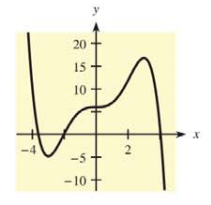Chapter 10.1, Problem 23E### Mathematical Applications for the ...

11th Edition
Ronald J. Harshbarger + 1 other
ISBN: 9781305108042

#### Solutions

Chapter
Section### Mathematical Applications for the ...

11th Edition
Ronald J. Harshbarger + 1 other
ISBN: 9781305108042
Textbook Problem

# For each function and graph in Problems 21-24(a) use the graph to identify x-values for which y ' >   0 , y ’ <   0 , y ' =   0 , and y’ does not exist.(b) use the derivative to check your conclusions. y = 6 + x 3 − 1 15 x 5(a)

To determine

The x-values where y>0,y<0 and y=0 for the function y=6+x3115x5 using the graph:Explanation

Given Information:

The provided function is y=6+x3115x5.

Explanation:

The critical values are the only values at which the graph can have turning points, the derivative cannot change sign anywhere except at the critical value.

This, in an interval between two critical values, the sign of the derivative at any value in the interval will be the sign of the derivative at all values in the interval.

As per the First Derivative Test,

The first derivative of the function is evaluated. The first derivative is made equal to zero in order to get the critical points.

The values of the critical values are kept inside the original function which gives the critical points. The intervals of the values of x are then evaluated for the relative maximum and minimum.

To obtain the relative maxima and minima of a function,

1. Find the first derivative of the function.

2. Set the derivative equal to 0 to obtain the critical points.

3. Evaluate f(x) at values of x to the left and one to the right of each critical point to develop a sign diagram.

(a) If f(x)>0 to the left and f(x)<0 to the right of the critical value, the critical point is a relative maximum point

(b)

To determine

To calculate: The conclusions from the derivative where the function y=6+x3115x5.

### Still sussing out bartleby?

Check out a sample textbook solution.

See a sample solution

#### The Solution to Your Study Problems

Bartleby provides explanations to thousands of textbook problems written by our experts, many with advanced degrees!

Get Started

#### Find more solutions based on key concepts